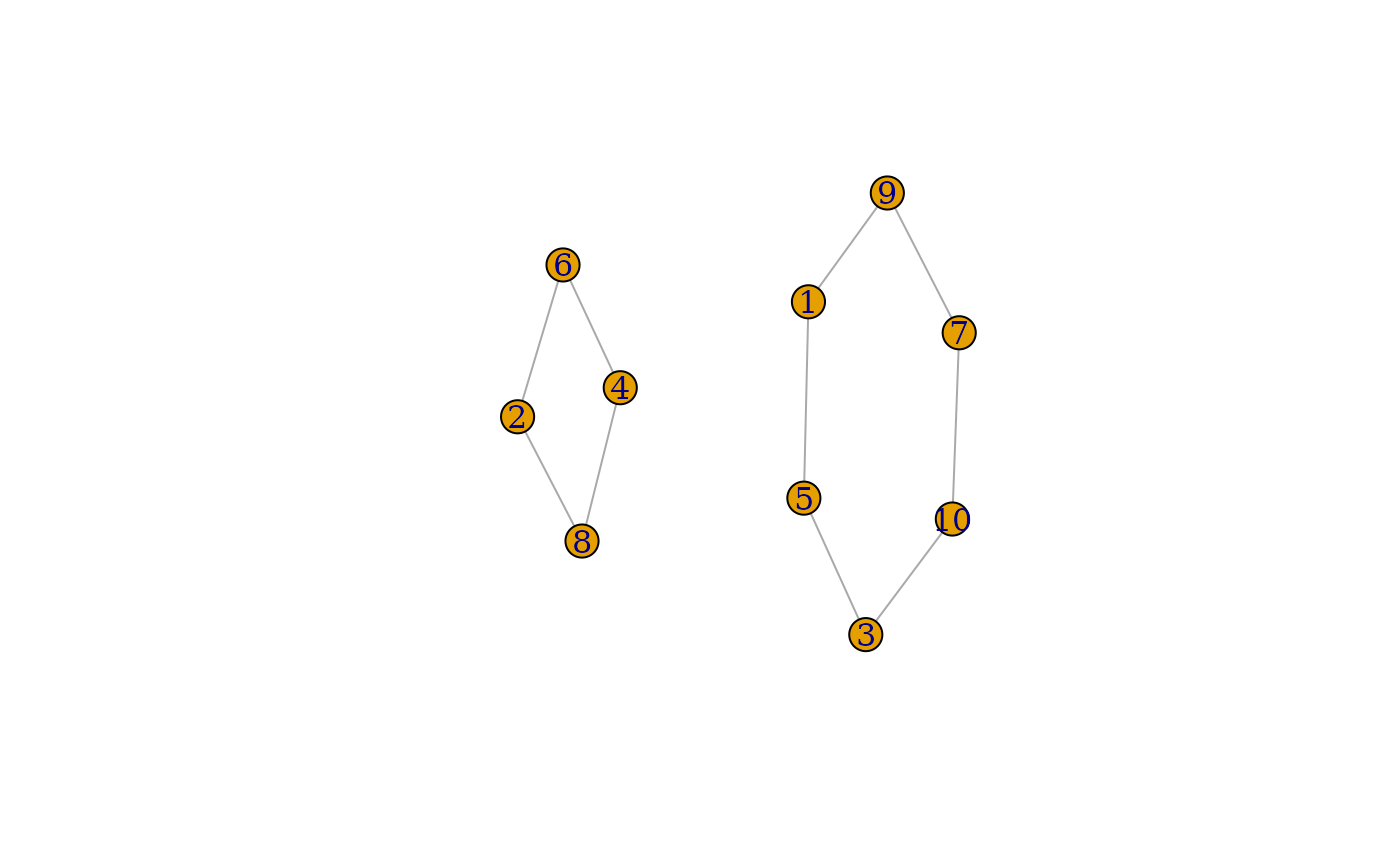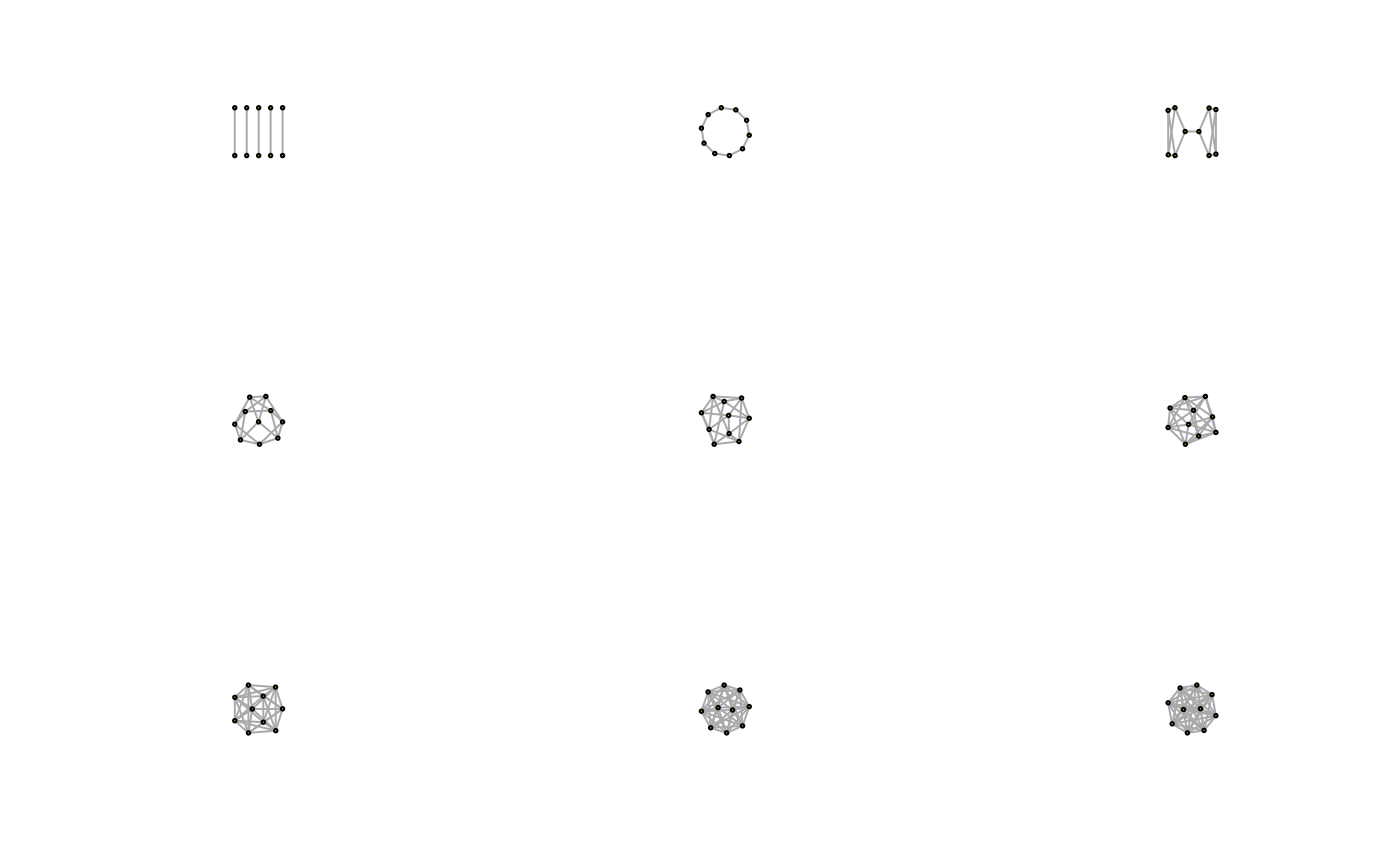Generate a random graph where each vertex has the same degree.

## Usage

sample_k_regular(no.of.nodes, k, directed = FALSE, multiple = FALSE)

## Arguments

no.of.nodes

Integer scalar, the number of vertices in the generated graph.

k

Integer scalar, the degree of each vertex in the graph, or the out-degree and in-degree in a directed graph.

directed

Logical scalar, whether to create a directed graph.

multiple

Logical scalar, whether multiple edges are allowed.

An igraph graph.

## Details

This game generates a directed or undirected random graph where the degrees of vertices are equal to a predefined constant k. For undirected graphs, at least one of k and the number of vertices must be even.

The game simply uses sample_degseq() with appropriately constructed degree sequences.

sample_degseq() for a generator with prescribed degree sequence.

Random graph models (games) erdos.renyi.game(), sample_bipartite(), sample_correlated_gnp_pair(), sample_correlated_gnp(), sample_degseq(), sample_dot_product(), sample_fitness_pl(), sample_fitness(), sample_forestfire(), sample_gnm(), sample_gnp(), sample_grg(), sample_growing(), sample_hierarchical_sbm(), sample_islands(), sample_last_cit(), sample_pa_age(), sample_pa(), sample_pref(), sample_sbm(), sample_smallworld(), sample_traits_callaway(), sample_tree(), sample_()

## Author

Tamas Nepusz ntamas@gmail.com

## Examples


## A simple ring
ring <- sample_k_regular(10, 2)
plot(ring)## k-regular graphs on 10 vertices, with k=1:9
k10 <- lapply(1:9, sample_k_regular, no.of.nodes = 10)

layout(matrix(1:9, nrow = 3, byrow = TRUE))
sapply(k10, plot, vertex.label = NA)#> []
#> NULL
#>
#> []
#> NULL
#>
#> []
#> NULL
#>
#> []
#> NULL
#>
#> []
#> NULL
#>
#> []
#> NULL
#>
#> []
#> NULL
#>
#> []
#> NULL
#>
#> []
#> NULL
#>